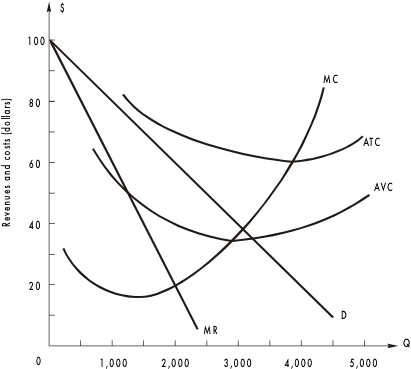# 100МС80ATC60AVC4020DMR1,0002,0003,0004,0005,000ioaso puD sonuoA

Question
19 views

Notes for graph: MC is marginal cost, MR is marginal revenue, ATC is average total cost, AVC is average variable cost and D is the demand curve.

1. To maximize the profit, how many units should the firm produce? At what price?
2. Based on your answer, what is the total revenue? Total costs? Total profit? Total fixed cost?
3. Will you operate this firm in the short run? Long run? Briefly explain.help_outlineImage Transcriptionclose100 МС 80 ATC 60 AVC 40 20 D MR 1,000 2,000 3,000 4,000 5,000 ioaso puD sonuoA fullscreen
check_circle

Step 1

1. In the aforementioned diagram, the downward sloping demand curve (D) and the marginal revenue (MR) curve are most important tools to determine the quantity of units produced and price charged by equating the marginal revenue curve with the marginal cost curve where the consumers’ willingness to pay (Price) will be determined by MR = MC and the ‘D’ curve. Thus, here, to maximize the profit 2, 000 units will be produced at price \$60.

Step 2

2.Further,

Total Revenue (TR) = Price (P) * Quantity produced (Q)

i.e., TR = \$60 * 2, 000

i.e., TR = \$1, 20, 000.

Total Cost (TC) = MC * Q

i.e., TC = \$20 * 2, 000

i.e., TC = \$40, 000.

Hence, Total Profit (TP) = TR – TC

i.e., TP = \$1, 20, 000 - \$40, 000

i.e., TP = \$80, 000.

However, Total Fixed Cost (TFC) can be determined by taking t...

### Want to see the full answer?

See Solution

#### Want to see this answer and more?

Solutions are written by subject experts who are available 24/7. Questions are typically answered within 1 hour.*

See Solution
*Response times may vary by subject and question.
Tagged in